Jet Set Go! All about Aeroplanes Jet Set Go! All about Aeroplanes

# Zero order Reaction Questions

A zero-order reaction is a chemical reaction in which the rate does not vary with the concentration of the reactants. As a result, the rate constant of the specific reactions is always equal to the rate constant of these reactions. Zero order reactions are relatively uncommon but they occur under special conditions. Some enzyme catalysed reactions and reactions which occur on metal surfaces are a few examples of zero order reactions.

 Definition: A reaction is said to be of zero order if its rate is independent of reactant concentration, i.e., the rate is proportional to the zeroth power of reactant concentration.

## Zero Order Reaction Chemistry Questions with Solutions

Q-1: A zero order reaction in A has a rate constant of 0.003 molL-1sec-1. How long will it take for A’s initial concentration to fall from 0.10 M to 0.075 M?

Answer: According to zero order reaction

[A] = [AO]-kt

Where [A] is the concentration at time, t

[AO] is the concentration at time = 0

k is the rate constant

Given: [A] = 0.075 M, [AO] = 0.10 M , k = 0.003 molL-1sec-1

Time,t=?

Substitute the values in the formula of zero order reaction

0.075 = 0.10 -0.003t

t= 8.33 s

Q-2: The half life of a zero order reaction is given by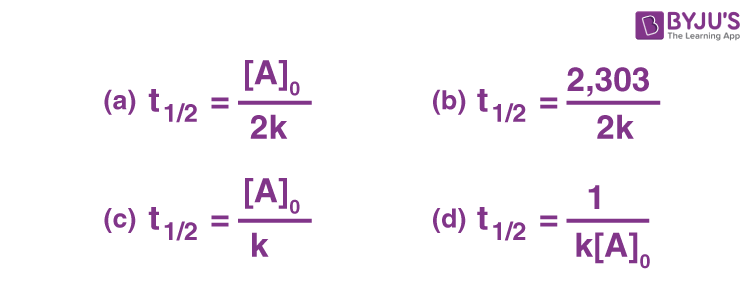Explanation: The expression for for a zero order rate constant is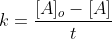Substituting t = t1/2, at which point [A] = [AO]/2 (at the half-life of a reaction, reactant concentration is half of the initial concentration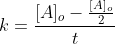Rearranging the equation, the expression for the half-life of a zero-order reaction is found to be:

t1/2 = [AO]/2k

Q-3: A radioactive decay is always considered as

1. Zero order reaction
2. First order Reaction
3. Both the above
4. Second order reaction

Q-4: Which of the following statements is(are) correct for zero order reactions?

1. Rate is independent of time
2. Reactants do not react with each other
3. One reactant is present in excess
4. Concentration of reactant decreases with time

Explanation: Rate= k(rate constant) for a zero order reaction. It means that it is independent of reactant concentration as well as time.

In addition, for zero order reactions, the concentration of the reactant decreases with time.

.

Q-5: Give some examples of zero order reactions.

Answer: Following are some examples of zero order reactions:

1) H2(g) + Cl2(g) 2HCl (g)

2)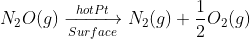3)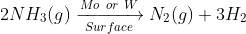Q-6: Which curve represents the zero order reaction.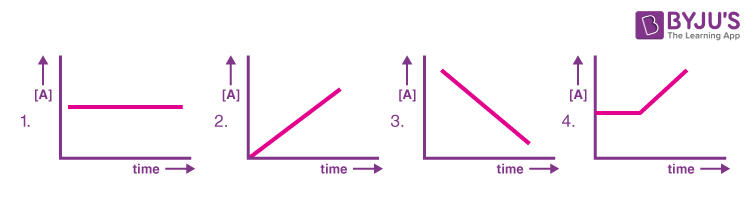Explanation: For a zero order reaction, concentration of A decreases with time as per the equation, [A] = [AO]-kt

Q-7: Consider a reaction A→B+C. If the initial concentration of A was reduced from 4M to 2M in 1 hour and from 2M to 1M in 0.5 hours, the order of the reaction is

1. Second
2. First
3. Third
4. Zero

Q-8: Match the graphical study with the order of the reactions: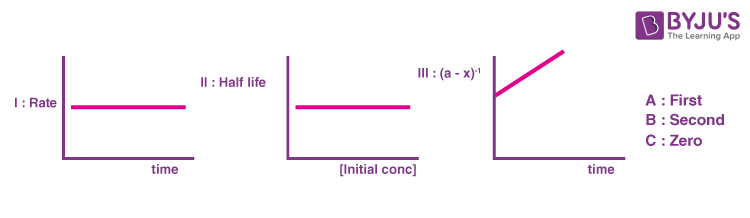Explanation: Because the rate of a zero order reaction is independent of time, Graph 1 depicts a zero order reaction.

The half life of a first order reaction is 0.693/k. It clearly demonstrates that it is unaffected by the initial concentration of reactant. As a result, graph 2 is the proper representation for first order reactions.

Hence, Graph III represents second order reaction.

Q-9: Choose the correct statement from U, V, or W for zero order reaction.

U: Unit of k is molL-1time-1

V: Time taken for 100% completion of reaction is [A]o/k.

W: The amount of the formed product is directly proportional to time.

Q-10: If the zero order reaction takes 100 min for 50% completion, how much time will it take for the 75% completion of the reaction?

For a zero order reaction, [A] = [AO]-kt

For 50% completion, [A] = 50 M, [AO]= 100 M, t50%= 100 min

[A] = [AO]- kt

50= 100-k(100)

k= 0.5 molL-1min-1

For 75% completion, [A] = 25 M, [AO]= 100 M, t75%= ?

[A] = [AO]-kt

25= 100- 0.5t

t= 150 min

Q-11: Zero order reactions has the same unit of rate constant and

1. Time
2. Reactant concentration
3. Rate
4. None of the above

Explanation: Rate law is defined for a zero order reaction with rate constant,k as

Rate = k[Reactant]O

Rate = k

Rate is defined as the change in reactant concentration over time. As a result, its unit will be molL-1s-1.

Because we can see Rate = k. As a result, the unit of rate constant, k will also be molL-1s-1.

Q-12: What is the rate equation for the reaction 2A+B→C if the order of the reaction is zero?

Answer: Since the order of reaction is zero, therefore rate is independent of reactant concentration. The rate equation will be, Rate=k[A]0[B]o.= k

Q-13: The decomposition of NH3 on the platinum surface is zero order reaction.Calculate the rates of production of N2 and H2 if k= 2.5×10-4 mol/L s.

Answer: The decomposition reaction of ammonia is: 2NH3→ N2 +3H2

For a zero order reaction, Experimental Rate = k[NH3]o = k

Theoretical Rate= -½ d[NH3]/dt=+d[N2]/dt =+ ⅓ d[H2]/dt

We know that theoretical rate is always equal to experimental rate, therefore,

Rate of production of N2= d[N2]/dt = k= 2.5×10-4 mol/L s

Rate of production of H2= d[H2]/dt = 3×k = 3×2.5×10-4 = 7.5 ×10-4 mol/L s

Q-14: k for a zero order reaction is 2×10-2 mol/L s. If the concentration of the reactant after 25s is 0.5 M. Calculate the initial concentration of the reactant.

Given:

Concentration of reactant after 25 s,[A] = 0.5 M= 0.5 mol/L

Rate constant, k = 2×10-2 mol/L s

Concentration of reactant initially, [AO]=?

The expression for a zero order reaction is given by

[A] = [AO] – kt

0.5 mol/L = [AO] -[(2×10-2 mol/L s) (25s)]

0.5 mol/L = [AO] -5×10-1 mol/L

[AO]= 1 mol/L

Q-15: Given the following observations:

Experiment [A] (mol/L) [B] (mol/L) Initial rate (mol/L min)
I 0.1 0.1 2×10-2
II X 0.2 4×10-2
III 0.4 0.4 Y

The reaction between A and B is first order in terms of A and zero order in terms of B. Determine the values of X and Y.

Answer: According to the given data, Rate = k[A][B]o= k[A]

Taking ratio of rates of experiment 1 and 2, we get

3)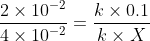On solving, X = 0.2 mol/L

Taking ratios of rates of experiment 1 and 3

3)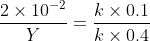On solving, Y= 0.08 mol/L

## Practise Questions on Zero Order Reaction

Q-1: For a zero order reaction, the half life depends on the initial concentration [Co] of the reactant as

a) [Co]

b) [Co]o

c) [Co]-1

d) [Co]½

Q-2: 3A → B+ C

It would be a zero order reaction when:

a) the reaction rate is proportional to the square of concentration of A.

b) the reaction rate remains the same at any concentration of A.

c) the reaction rate remains unchanged at any concentration of B and C.

d) the reaction rate doubles if concentration of B is increased to double.

Q-3: Calculate the time for the reaction to complete if “a” is the initial concentration of the substance that reacts according to zero order kinetics and “k” is the rate constant.

Q-4: The half life period of a specific reaction A→ Products is one hour. If reactant ‘A’ has an initial concentration of 2 mol/L, how long does it take for its concentration to decrease from 0.50 to 0.25 mol/L in a zero order reaction?

Q-5: For a general reaction, A→C, the plot of concentration of A versus time is given in the figure below. Find the slope and intercept of the curve.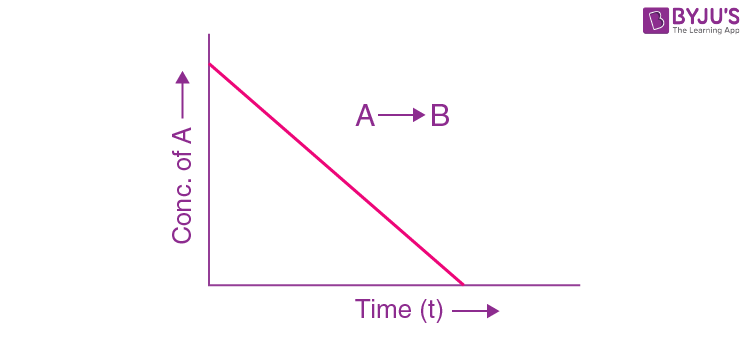Click the PDF to check the answers for Practice Questions.

## Zero Order Reaction